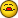# Conditional Identifiaction Of Unique Entries

I

#### IanEmery

Hi

I formula that identifies unique entries in a column and counts the
total as shown below:

=SUMPRODUCT(B8:B501<>"")/(COUNTIF(B8:B501,B8:B501&"")))

I am trying to get this formula to work only where a precondition
exists, i.e. that an associated field is set to "yes"

This works fine on normal SUMIF functions as shown below:

=SUMIF(S8:S501,"Yes",P8525)

However when I try o combine the 2 scenarious I receive a Value#
error.

The formula I am trying is shown below:

=IF(S8:S501,"Yes",SUMPRODUCT(B8:B501<>"")/(COUNTIF(B8:B501,B8:B501&"")))

Anyone any ideas?

Many thanks

S

#### SteveG

Not sure if this is exactly what you want because your SUMIF refers to
P8501 and your SUMPRODUCT does not but this might get you there,

=SUMPRODUCT(--(S8:S501="Yes"),--(B8:B501<>""),P8501)/COUNTIF(B8:B501,B8:B501&"")

HTH

Stev

I

#### IanEmery

Hi Steve

I have changed the formula to reflect the correct field references aa
shown below but receive a #DIV/0! error:

=SUMPRODUCT(--(S8:S501="Yes"),--(A8:A501<>""),A8:A501)/COUNTIF(A8:A501,A8:A501&"")

Can you see any problems ?

Thank

G

#### Guest

=SUMPRODUCT(--(S8:S501="Yes"),--(A8:A501<>""),(A8:A501))/COUNTIF(A8:A501,A8:A501&"")

It's work here, if you can send a sample of the type of data you have in
column A and S

HTH
Regards from Brazil
Marcelo

"IanEmery" escreveu:

S

#### SteveG

Ian,

I think this is what you are looking for.

=SUMPRODUCT(--(S8:S501="Yes"),--(A8:A501<>""),A8:A501)/SUMPRODUCT((A8:A501<>"")/COUNTIF(A8:A501,A8:A501&""))

This will sum A8:A501 IF S8:S501 = "Yes" AND IF A8:A501 is not blank
and divide by the number of unique values excluding blanks in A8:A501.

Does that work for you?

Steve

I

#### IanEmery

Thanks for the formula.

This is returning a count of 0 with the following data extract:

Range A8:A501 contain numbers of which are shown below:

A8:1
A9:1
A10:2
A11:2
A12:1
A13:3
A14:4

Range S8:S501 contains a formula which dreives a Yes or Null value as
shown below:

S8:
S9:Yes
S10:Yes
S11:Yes
S12:
S13:Yes
S14:

As such the count should return a result of 3

The formula to derive the Yes value is shown below for information:

=IF(R9 > \$H\$2,"Yes",IF(M9 > R9, "Yes", ""))

Any further assistance would be much appreciated

S

#### SteveG

Ian,

=SUM(IF(FREQUENCY(IF((A8:A501<>"")*(S8:S501="Yes"),MATCH(A8:A501,A8:A501,0)),ROW(INDIRECT("1:"&ROWS(A8:A501))))>0,1))

This is an array formula so commit with Ctrl-Shift-Enter.

Credit Bob Phillips for this one. I dug this out of one of hi
previous posts for you.

HTH

Stev